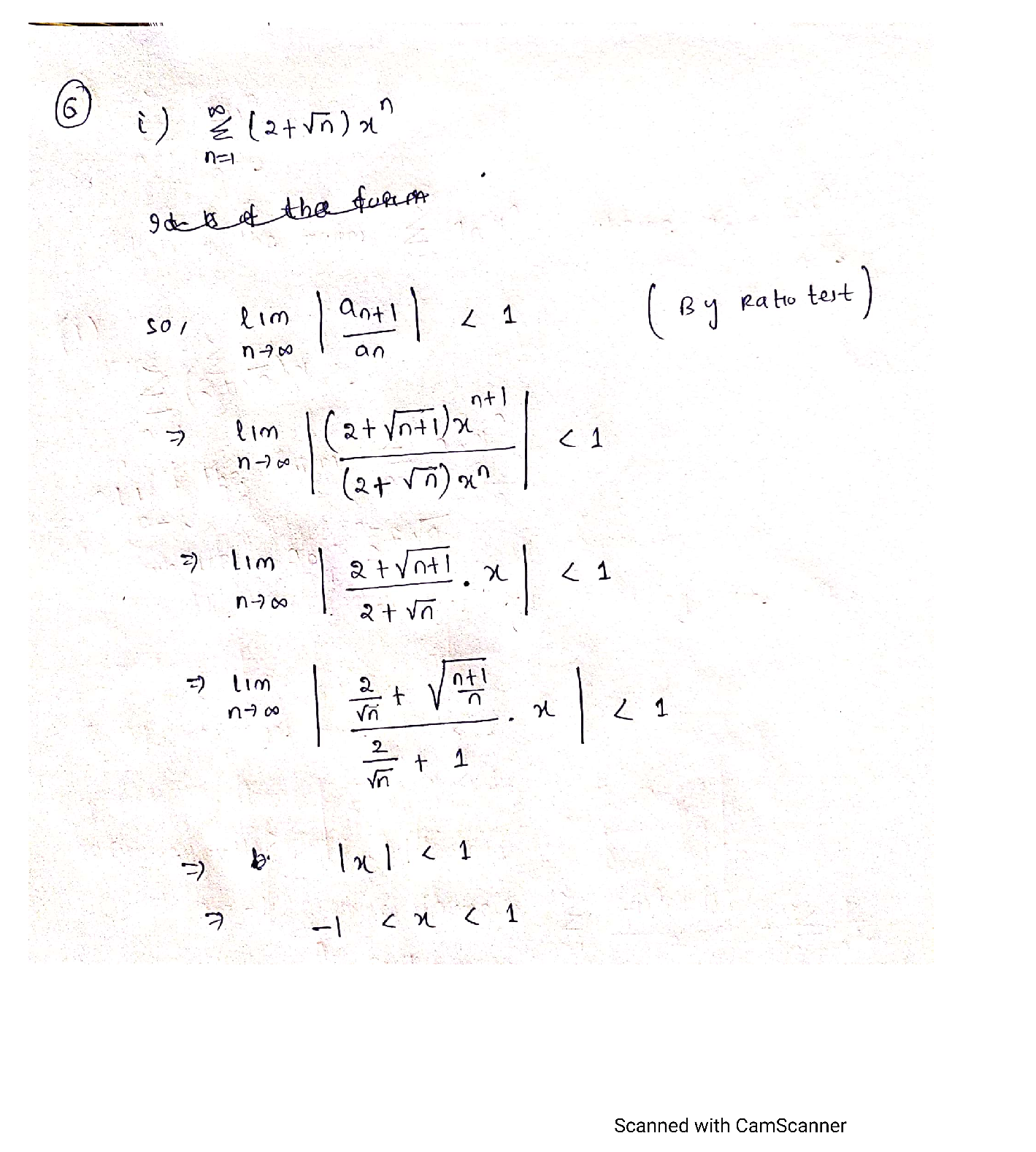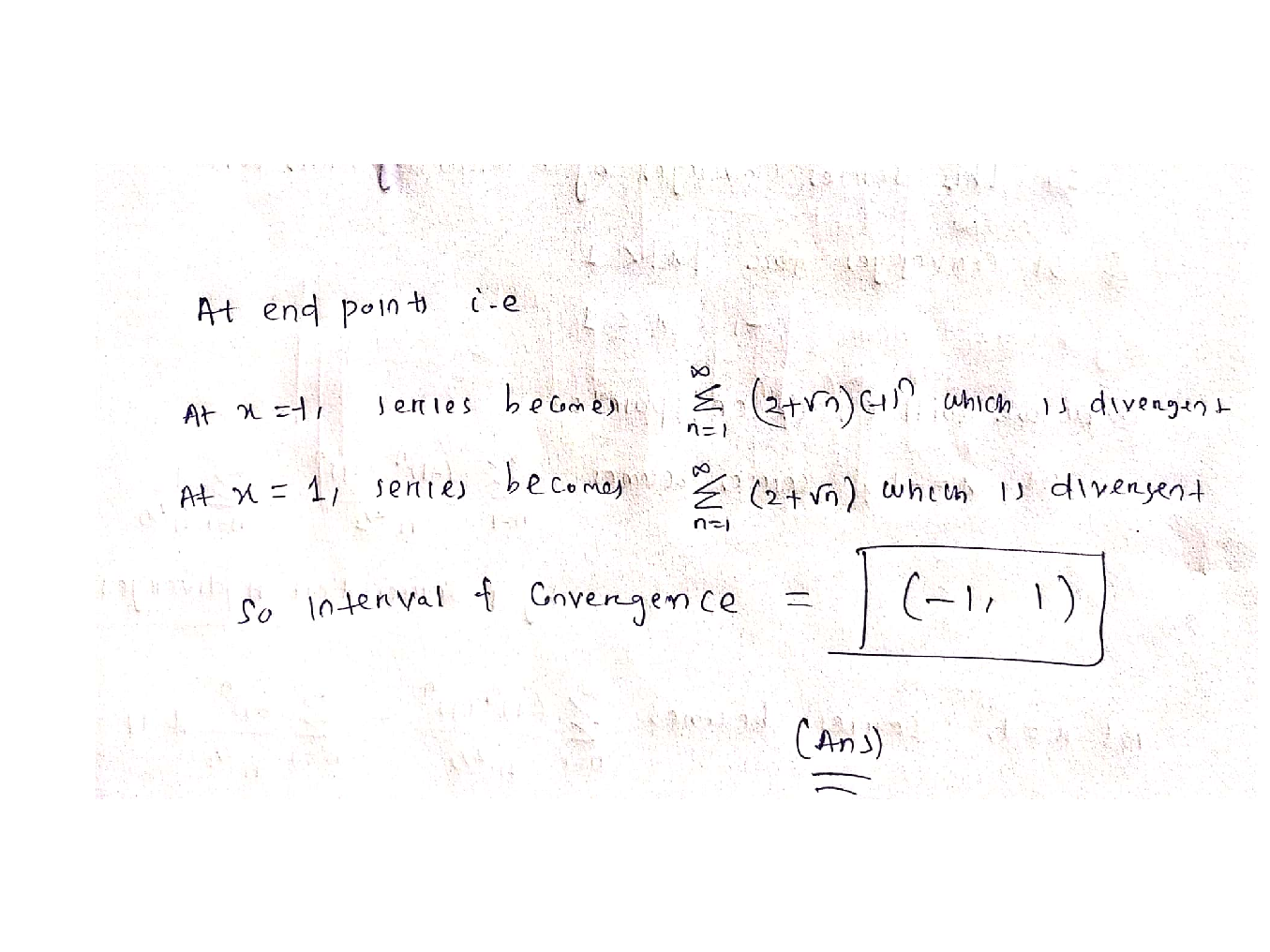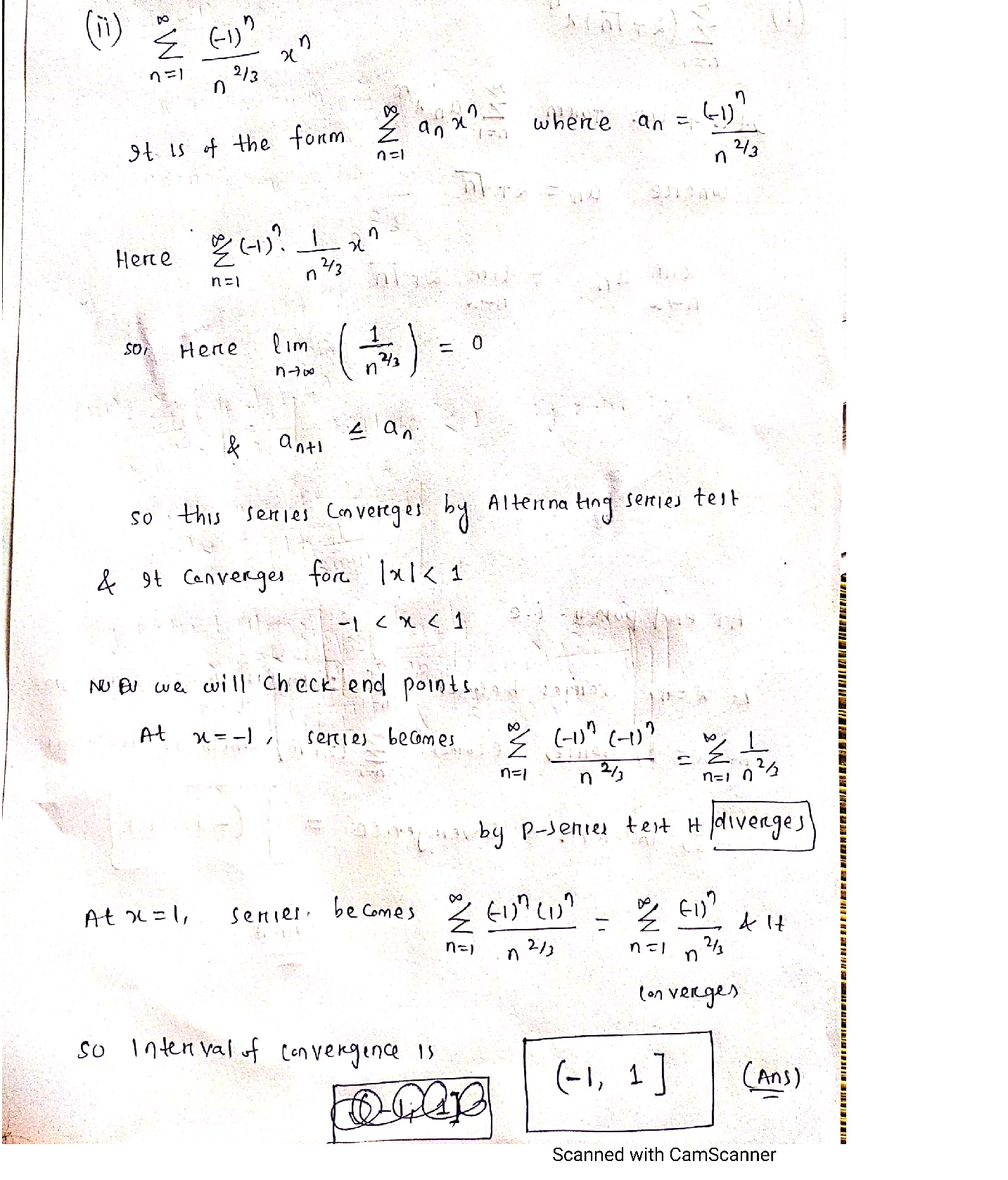Question

Sequence and Series

\text { Define the sequence }\left(a_{n}\right)_{n \geq 1} \text { recursively by } a_{1}=1 \text { and } a_{n+1}=\sqrt{2+a_{n}} \text { for all } n \geq 1

\text { i. Prove that for all } n \geq 1 \text { we have } 1 \leq a_{n} \leq 2

\text { ii. Prove that }\left(a_{n}\right)_{n \geq 1} \text { is a monotonic sequence. }

iii. Deduce that the sequence converges with limit 2.

iv. Show that for all n > 1 we have

2-a_{n} \leq \frac{1}{(2+\sqrt{3})^{n-1}}Verified### Question 41232Sequence and Series

5: Determine whether the alternating series converges.
\sum_{n=1}^{\infty}(-1)^{n+1} \frac{1}{n^{2}}

### Question 41231Sequence and Series

Example 5: Show that the ratio test is inconclusive for the following series. Then use another method to determine the convergence or divergences of the series.
\sum_{n=1}^{\infty} \frac{n^{2}+1}{(n+1)^{2}}

### Question 41230Sequence and Series

\sum_{n=1}^{\infty} \frac{n^{2}-4}{2^{n}}
Use the ratio test to determine whether the series converges or diverges.

### Question 41229Sequence and Series

Example 3: Use any test covered in 11.1-11.6 to determine whether the following seriesconverges ordiverges.
\sum_{n=1}^{\infty} \frac{1-\sin n}{n^{3 / 2}}

### Question 41228Sequence and Series

Example 2: Use the limit comparison test to determine whether the following series converges or diverges.
\sum_{n=1}^{\infty} \frac{n^{2}-2 n+5}{\sqrt{3+n^{5}}}

### Question 41227Sequence and Series

Example 1: Use the comparison test to determine whether the following series converges or diverges.
\sum_{n=1}^{\infty} \frac{\ln n}{n}

### Question 39894Sequence and Series

Determine the Taylor series of the given function. Find the radius of convergence.
f(x)=\cos \left(2 x^{3}\right)

### Question 39893Sequence and Series

Find the power series for the given function. What is the radius of convergence?
f(x)=\frac{1}{1+3 x}

### Question 39892Sequence and Series

Determine the interval of convergence for the given power series.
\sum_{n=0}^{\infty} \frac{(3 x)^{n}}{n !}

### Question 39891Sequence and Series

5: Decide whether the series converges. If so, find its sum.
\sum_{n=1}^{\infty} \frac{3}{n(n+1)}
\sum_{n=2}^{\infty} \frac{3^{n}-1}{5^{n-2}}
Use the Ratio or Root test, to determine whether the series converges or diverges.
\sum_{n=1}^{\infty} \frac{(\ln n)^{2 n}}{n^{n+1}}

### Submit query

Getting answers to your urgent problems is simple. Submit your query in the given box and get answers Instantly.

### Submit a new QuerySuccess

Assignment is successfully created